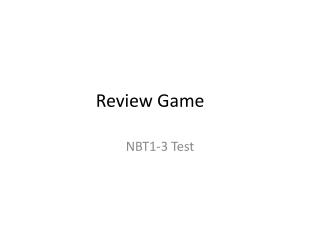DownloadDownload PresentationReview Game

# Review Game

Download Presentation## Review Game

- - - - - - - - - - - - - - - - - - - - - - - - - - - E N D - - - - - - - - - - - - - - - - - - - - - - - - - - -
##### Presentation Transcript

1. Review Game NBT1-3 Test

2. Compare <,>,= 9.873 ____ 9.863

3. Compare <,>,= • 753.5 ____ 753.50

4. Write the value for the expression • 1/1000 =

5. Base Ten • 10⁵

6. Base Ten • 10⁶

7. Fraction, Expanded Form, Standard Form • Fraction: 8/100 • Expanded: ______ • Standard: ______

8. Fraction, Expanded Form, Standard Form • Fraction: _____ • Expanded: ______ • Standard: 8.57

9. Fraction, Expanded Form, Standard Form • Fraction: ______ • Expanded: (5x100) + (4x1) +(5x1/10)+ (8x1/100) • Standard: ______

10. Fraction, Expanded Form, Standard Form • Fraction: _____ • Expanded: (8x1000) + (5x 10) + (8x1) + (5 x 1/100) • Standard: ______

11. Place Value • 4,859,732 • What place is the 8 in?

12. Place Value • 4,859,732 • What place is the 7 in?

13. Place Value • 4,859,732 • What place is the 4 in?

14. Place Value • 4,859,732 • What is the value of the 8 in?

15. Place Value • 4,859,732 • What digit is in the hundred thousand place?

16. Standard Form • 50,000+ 400+ 30+ 1

17. Standard Form • 300,000 + 9,000 + 70 + .5 + .08

18. Expanded Form (EF) Word Form (WF) • 585.87 EF____________________________ WF _______________________________

19. Expanded Form (EF) Word Form (WF) • 5785.458 EF _____________________ • WF_____________________